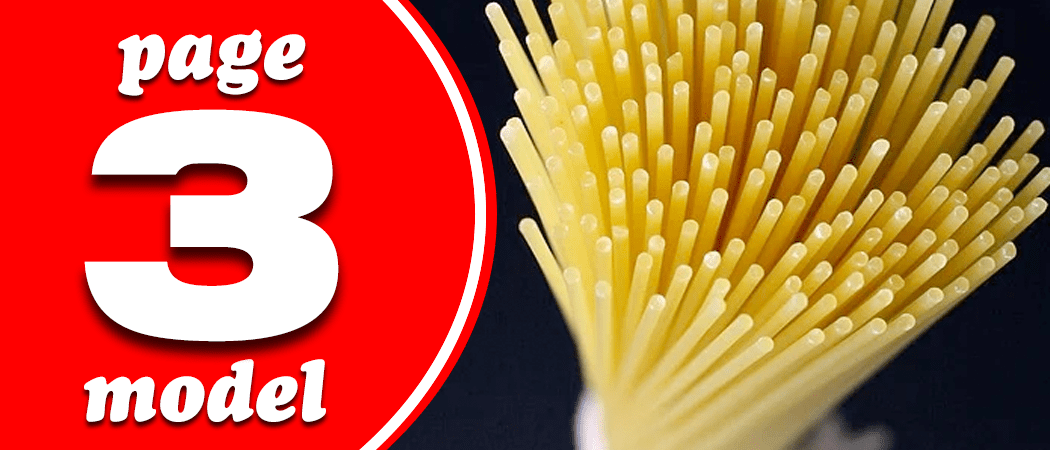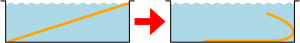# Page 3 model: Cooking spaghetti

Some saucy modellingYou there! Yes—you cooking the spaghetti! How do you tell when it’s cooked? No, don’t throw it against the wall… maths is here for you.

Cooking pasta increases the amount of water it contains until the pasta is fully hydrated, at which point we say it’s cooked. But this means that the pasta is no longer a rigid material—it’s flexible.

Nathaniel Goldberg and Oliver O’Reilly, in their 2020 paper, model the process of spaghetti cooking in a saucepan:Measure the arclength, $s$, of your spaghetto from $s=0$ to $s=L$ and call the angle to the horizontal at any point $\theta(s)$:Flexible rod theory tells you that the moment along the spaghetto, $\boldsymbol{m}$, depends on the angle, $\theta$, and the weight force, $\boldsymbol{n}$. For a bent spaghetto, you can write the curvature, $\kappa$, in terms of the intrinsic curvature, $\kappa_0$, and the moment, divided by the rigidity, $EI$: $\frac{\partial\theta}{\partial s} = \kappa = \kappa_0 + \frac{m}{EI}.$

## In the pan!

But the intrinsic curvature changes as water is absorbed. Goldberg & O’Reilly use a model from plant stem growth for the rate of intrinsic curvature change, making it dependent on the amount of time the spaghetto has been in the water for:

$\frac{\partial \kappa_0}{\partial t} = \alpha(t)(\kappa – \kappa_0) \quad \text{where} \quad \alpha(t) = \alpha_{\infty}\frac{1-\mathrm{e}^{-t/\tau}}{1+\mathrm{e}^{-(t-t_0)/\tau}}.$

Want the spaghetti al dente? Better get working out the parameters $\alpha_\infty$ and $\tau$…

## References

1. NN Goldbery & OM O’Reilly Mechanics-based model for the cooking-induced deformation of spaghetti, Physical Review E, 101, 03001, (2020).Eleanor is a postdoctoral researcher at the University of Manchester. A mathematician by training, she works on developing mathematical models to improve our understanding of biological mechanisms in medicine, with particular interests in women’s health and autoimmune conditions. When not doing mathematics, she crochets, sews and reads everything and anything.